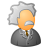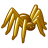New to Qlik Sense

If you’re new to Qlik Sense, start with this Discussion Board and get up-to-speed quickly.

Announcements
QlikWorld 2022, LIVE in Denver CO., May 16-19, 2022. REGISTER NOW TO RECEIVE EARLY BIRD PRICING
cancel
Showing results for
Did you mean:Contributor III

calculate correlation using variables Qlik Sense

Hi!

I have two variables defined in Qlik Sense variables editor:

NameDefinition
vVar1sum(Field1)
vVar2sum(Field2)/sum(Field3)

I need to create table like this:

DimensionCorrelation
Item 10.9
Item 20.6

How i can calculate correlation?

For now i am trying in expression editor :

RangeCorrel(\$(vVar1),\$(vVar2)), but result is "-" for every item.

What is wrong?

1 Solution

Accepted SolutionsPartner

Correl( Aggr( Sum(Field1) , Dimension) , Aggr( Sum(Field2)/Sum(Field3) , Dimension ) )

Aggr( <aggreation-expression> , Dimension) will return a number of results from the aggregation-expression grouped by Dimension. So if Dimension contains 4 distinct values then you will get the four return values. These will be returned to Correl() function as the set of values.

6 RepliesPartner

RangeCorrel() is a range function and as such will only accept a list of discrete values arranged as pairs or a list of values returned from the inter-record functions Above, Below, Before, After, Top, Bottom, First and Last.

It might be that the Correl() function is the one you should use since it takes bare fields as parameters - not including any aggregation function.

You might need to use the Aggr() function along with the Correl() if you need to use Sum() to sum up before you caluculate the correlation.Contributor III
Author

Please, can you give me example of formula how it could looks with Aggr() and Correl()?Partner

Correl( Aggr( Sum(Field1) , Dimension) , Aggr( Sum(Field2)/Sum(Field3) , Dimension ) )

Aggr( <aggreation-expression> , Dimension) will return a number of results from the aggregation-expression grouped by Dimension. So if Dimension contains 4 distinct values then you will get the four return values. These will be returned to Correl() function as the set of values.Contributor III
Author

I will give you more details: In data table i have customers, items, DateMY, salesSum, salesQuantity, salesProfit (customers, items, DateMY not unique values).

So what will be formula for table with first column as Customers and second column Correlation?

Is it correct

Correl( Aggr( Sum(salesQuantity) , DateMY, items) , Aggr( Sum(salesSum)/Sum(salesProfit) , DateMY, items) ) ?Partner

Correl( Aggr( Sum(salesQuantity) , DateMY, items) , Aggr( Sum(salesSum)/Sum(salesProfit) , DateMY, items) )

It Seems Ok did you try this  ?

Or

try below  expression in your chart with dimension

Correl(SalesWQuantity,salesSum/SalesProfit)

There is some Limitation with this function

The expression must not contain aggregation functions, unless these inner aggregations contain the TOTAL qualifier. For more advanced nested aggregations, use the advanced aggregation function Aggr, in combination with calculated dimensions.

https://help.qlik.com/en-US/qlikview/November2017/Subsystems/Client/Content/ChartFunctions/Statistic...

Regards

RamanContributor III
Author

Thanks.Tags
Community Browser